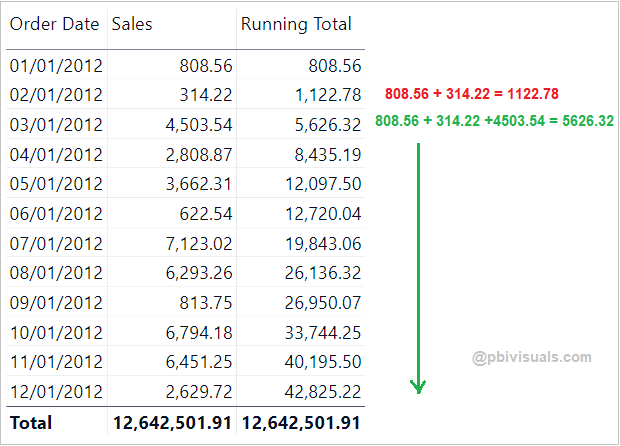Home » Power BI » Power BI Running Totals/ Cumulative Totals

# Power BI Running Totals/ Cumulative Totals

The Cumulative or Running total is used to watch the summation of numbers that is updated every time when a new number is entered to the sequence.

### Let’s get started-

Global_superstore_2016.xls

### Following these steps-

Step-1: Add one measure & write below DAX in order to create a running total-

```Running Total =

CALCULATE (
SUM(Orders[Sales]),
FILTER (
ALL(Orders[Order Date]),
Orders[Order Date] <= MAX(Orders[Order Date] )
)
)```

#### Measure Description:

• CALCULATE:  Evaluates an expression in a modified filter context.
• SUM: The SUM function is a aggregation function and it calculates the sum of all numbers in a column.
• FILTER: Returns a table that represents a subset of another table or expression.
• ALL: Returns all the rows in a table, or all the values in a column.
• MAX: Returns the largest value in a column.

Step-2: Now drag measure into table visual to see the running total result.Running Totals in Power BI

### You can also create a running total by using another DAX functions-

```DATESYTD =

CALCULATE(SUM(Orders[Sales]),

DATESYTD(Orders[Order Date])```DATESYTD DAX

It returns the year wise running total and for every year it will start sales summation from the beginning. In same way you can use DATESMTD & DATESQTD DAX functions to get the running totals.

Refer more Power BI Post – Power BI